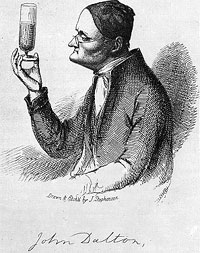# Who was John Dalton?From the Bruker Daltonics site:

John Dalton (1766-1844) of Manchester, England was one of the first persons to look at matter in relation to molecules and atoms. In the course of his studies and teaching, he developed the ideas which led to his formulation of the atomic theory of matter in 1805.

Dalton was the first to come up with a theory that attempted to explain the empirical (experimental or hypothetical) laws of nature pertaining to chemical measurements, especially those of mass. By attempting to explain these empirical laws, he developed the atomic theory of matter.

Probably the best-known and most widely accepted of the empirical laws known to Dalton was the law of conservation of (total) mass, which had been used by Lavoisier in 1798. The Law of Conservation of Mass can be stated as follows: The total mass of the reactants in any chemical reaction is exactly equal to the total mass of the products.

The second empirical law known to Dalton was the Law of Constant Composition of compounds (attributed to Proust, 1755 - 1826) which stated that any pure substance has a fixed composition in terms of the chemical elements.

In other words, for every pure compound the mass ratios of its constituent elements are constant and cannot be altered without changing the other properties which are also characteristic of the compound.

The empirical Law of Multiple Proportions was apparently developed by Dalton himself around 1804. The law of multiple proportions is as follows: When any two elements are observed to form more than one compound between them, the mass ratios in one compound will be related to the mass ratios in the other in the proportions of whole numbers.

How did he do it? The easiest way to determine mass ratios in compounds is by decomposition to the elements followed by elemental analysis. However, in the early nineteenth centuries, Bruker Daltonics had not yet started making mass spectrometers, so many of the ratios were instead determined by synthesizing compounds from pure elements.

For example, when a measured amount of hydrogen was burned in excess oxygen, an exact amount of water always evolved. The water product was weighed, and since the hydrogen used was known, the oxygen used was determined assuming conservation of mass. In this way it was found that water is 11.19% hydrogen and 88.81% oxygen by mass. Since it was the lightest element known, and assumed to be a fundamental value, Dalton assumed that hydrogen should have an atomic mass of 1.

The mass of oxygen was thus calculated using the ratio 88.81/11.19 = x/1, where x is the atomic mass of oxygen, and the value of 7.937 obtained was rounded to eight. While this answer is incorrect, it was a pretty good determination given the tools available at the time.

Today, units of atomic mass (atomic mass units or "amu's") are often referred to as "daltons" in honor of John Dalton. His analytical apparatus could appropriately be called the world's first mass spectrometer. Today, Bruker Daltonics honors John Dalton by producing the finest mass spectrometers in the world.